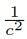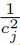## Abstract

Choosing the scale or shape parameter of radial basis functions (RBFs) is a well-documented but still an open problem in kernel-based methods. It is common to tune it according to the applications, and it plays a crucial role both for the accuracy and stability of the method. In this paper, we first devise a direct relation between the shape parameter of RBFs and their curvature at each point. This leads to characterizing RBFs to scalable and unscalable ones. We prove that all scalable RBFs lie in the-class which means that their curvature at the point xj is proportional to, where cj is the corresponding spatially variable shape parameter at xj. Some of the most commonly used RBFs are then characterized and classified accordingly to their curvature. Then, the fundamental theory of plane curves helps us recover univariate functions from scattered data, by enforcing the exact and approximate solutions have the same curvature at the point where they meet. This leads to introducing curvature-based scaled RBFs with shape parameters depending on the function values and approximate curvature values of the function to be approximated. Several numerical experiments are devoted to show that the method performs better than the standard fixed-scale basis and some other shape parameter selection methods.

How to Cite
Heidari, M., Mohammadi, M., & De Marchi, S. (2023). Curvature based characterization of radial basis functions: application to interpolation. Mathematical Modelling and Analysis, 28(3), 415–433. https://doi.org/10.3846/mma.2023.16897
Published in Issue
Sep 4, 2023
Abstract Views
64
67

## References

M. Bozzini, L. Lenarduzzi, M. Rossini and R. Schaback. Interpolation with variably scaled kernels. IMA Journal of Numerical Analysis, 35(1):199–219, 2015. https://doi.org/10.1093/imanum/drt071

M. Bozzini, L. Lenarduzzi and R. Schaback. Adaptive interpolation by scaled multiquadrics. Advances in Computational Mathematics, 16(4):375–387, 2002. https://doi.org/10.1023/A:1014584220418

G.E. Fasshauer. Meshfree approximation methods with MATLAB. World Scientific, 2007.

G.E. Fasshauer and M.J. McCourt. Kernel-based approximation methods using MATLAB. World Scientific Publishing Company, 2015. https://doi.org/10.1142/9335

B. Fornberg and J. Zuev. The Runge phenomenon and spatially variable shape parameters in RBF interpolation. Computers & Mathematics with Applications, 54(3):379–398, 2007. https://doi.org/10.1016/j.camwa.2007.01.028

B. Fornberg and J. Zuev. The Runge phenomenon and spatially variable shape parameters in RBF interpolation. Computers & Mathematics with Applications, 54(3):379–398, 2007. https://doi.org/10.1016/j.camwa.2007.01.028

M. Heidari, M. Mohammadi and S. De Marchi. A shape preserving quasiinterpolation operator based on a new transcendental RBF. Dolomites Research Notes on Approximation, 14(1):56–73, 2021. https://doi.org/10.14658/pupj-drna-2021-1-6

E.J. Kansa. Multiquadrics-A scattered data approximation scheme with applications to computational fluid-dynamics-II solutions to parabolic, hyperbolic and elliptic partial differential equations. Computers & Mathematics with applications, 19(8-9):147–161, 1990. https://doi.org/0.1016/0898-1221(90)90271-K

E.J. Kansa and R.E. Carlson. Improved accuracy of multiquadric interpolation using variable shape parameters. Computers & Mathematics with Applications, 24(12):99–120, 1992. https://doi.org/10.1016/0898-1221(92)90174-G

L. Ling. A univariate quasi-multiquadric interpolation with better smoothness. Computers & Mathematics with Applications, 48(5):897–912, 2004. https://doi.org/10.1016/j.camwa.2003.05.014

B. Óneill. Chapter 7 - Riemannian geometry. In Elementary Differential Geometry (Second Edition), pp. 321–387. Academic Press, Boston, 2006. https://doi.org/10.1016/B978-0-12-088735-4.50011-0

R.S. Palais. A modern course on curves and surfaces. Virtual Math Museum, 2011. Available from Internet: https://virtualmathmuseum.org/Surface/a/bk/curves_surfaces_palais.pdf

H. Ratliff. Cartesian formulas for curvature, circumradius, and circumcenter for any three two-dimensional points. Zenodo, 2019. https://doi.org/10.5281/zenodo.2556424

M. Rossini. Interpolating functions with gradient discontinuities via variably scaled kernels. Dolomites Research Notes on Approximation, 11(2):3–14, 2018. https://doi.org/10.14658/pupj-drna-2018-2-2

S.A. Sarra and D. Sturgill. A random variable shape parameter strategy for radial basis function approximation methods. Engineering Analysis with Boundary Elements, 33(11):1239–1245, 2009. https://doi.org/10.1016/j.enganabound.2009.07.003

R. Schaback. Error estimates and condition numbers for radial basis function interpolation. Advances in Computational Mathematics, 3(3):251–264, 1995. https://doi.org/10.1007/BF02432002

A.K. Singh and B.S. Bhadauria. Finite difference formulae for unequal subintervals using Lagrange’s interpolation formula. International Journal of Mathematical Analysis, 3(17):815–827, 2009.